## An electron is accelerated through a potential difference of 2.8 kV and directed into a region between two parallel plates separated by 17 m

Question

An electron is accelerated through a potential difference of 2.8 kV and directed into a region between two parallel plates separated by 17 mm with a potential difference of 100 V between them. The electron is moving perpendicular to the electric field when it enters the region between the plates. What magnetic field is necessary perpendicular to both the electron path and the electric field so that the electron travels in a straight line

in progress 0
2 months 2021-07-31T05:23:16+00:00 2 Answers 3 views 0

B=1.89*10^{-4} T

Explanation:

First we have to calculate the electric force on the electron, and then we have to take into account that this force is equal to the force generated by the magnetic field.

The formula is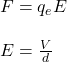q: charge of the electron = 1.6*10^{-19}C

V: potential

d: separation between plates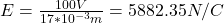This force must equal the Lorentz’s force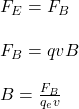But before we have to calculate the speed of the electron by using  (me=9.1*10^{-31}kg)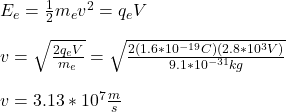Hence, we have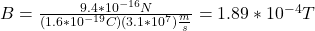hope this helps!!

The magnetic field that is necessary is equal to 1.88×10⁻⁴ T

Explanation:

If the electron is accelerated: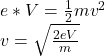Where

e = 1.6×10⁻¹⁹C

V = 2.8 kV = 2800 V

m = 9.1×10⁻³¹kg

Replacing: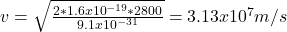When the electron is moving in straight line, the magnetic force is balanced with the electric force, thus:

V = E * d

Where V = 100 V

d = 17 mm = 0.017 m

E = V/d = 100/0.017 = 5882.35 N/C

The magnetic field that is necessary is equal to:

B = E/v = 5882.35/3.13×10⁷ = 1.88×10⁻⁴ T